# 1st PUC Physics Question Bank Chapter 4 Motion in a Plane

Students can Download Physics Chapter 4 Motion in a Plane Questions and Answers, Notes Pdf, 1st PUC Physics Question Bank with Answers helps you to revise the complete Karnataka State Board Syllabus and to clear all their doubts, score well in final exams.

## Karnataka 1st PUC Physics Question Bank Chapter 4 Motion in a Plane

Scalars and Vectors

Question 1.
What is a scalar quantity?
A physical quantity which has only magnitude but no direction is called a scalar quantity.

Question 2.
Give few examples for scalar quantity.
Distance travelled, mass, time, temperature etc,.Question 3.
Yes, scalar obeys the ordinary addition rules. Scalars can be added, subtracted multiplied and divided just as the ordinary numbers.

Question 4.
What is vector quantity?
A Physical quantity which has both magnitude and direction is called a vector quantity.

Question 5.
Give few examples for vectors.
Displacement, velocity, acceleration, force etc,

Question 6.
Pick out the only vector quantity in the following list:
temperature, pressure, impulse, path length and energy.
Impulse

Question 7.
Pick out the scalar quantity from the following quantities area, displacement distance travelled, momentum.
Distance travelled.

Question 8.
Does the scalar addition obey ordinary addition rules? Explain with an example.
Yes scalar obey ordinary addition rules.
Example – If the length and breadth of a rectangle are 0.1 m and 0.5 m respectively, then its perimeter is sum of the length of four sides, i.e., perimeter = 1 + 0.5 + 1 + 0.5 = 3m. The length of each sides is scalar and perimeter is also scalar.

Question 9.
Give an example of a physical quantity which can be treated has both scalar and vector?
Surface area.

Question 10.
Even though electric current has direction, it is not a vector. Why?
Because it does not obey the laws of vector addition.Question 11.
How is a vector represented?
A vector is represented by a straight line with an arrowhead. The length of the line represents the magnitude and the arrowhead represents the direction.

Question 12.
What is position vector?
A vector which gives the position of a particle with reference to the origin of a co-ordinate system is called position vector.
Let O be the origin of the co-ordinate system. Let P be the position of a particle at any instant of time. The $$\overrightarrow{O P}=\vec{r}$$ is the position vectors.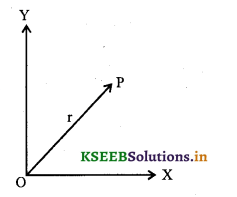Question 13.
Is displacement a scalar or a vector?
Displacement is a vector quantity.

Question 14.
What is displacement vector?
It is a straight line joining the initial and final position of a particle.

Question 15.
Explain the term displacement vector.
Let O be the origin of a coordinate system. Let P and P’ be the position of a particle of a particle at time t and t’ respectively. If the particle move from P to P’, PP’ is called displacement vector.
Displacement vector = $$\overrightarrow{P P^{\prime}}$$.Question 16.
Can the magnitude of displacement is greater than the path length?
No, the magnitude of displacement is either less or equal to path length of an object between two points.

Question 17.
When two vector are said to be equal? OR What are equal vectors?
Two vectors are said to be equal, if and only if, they have same magnitude and the same direction.

Question 18.
Explain the method to check the equality of two vectors.
Consider two vectors A and B represented by OP and QS both is magnitude and direction. Shift the vector B such that its tail Q coincides with O and tip S coincides write P. Then the two vectors said to be equal vectors i.e., $$\vec{A}=\vec{B}$$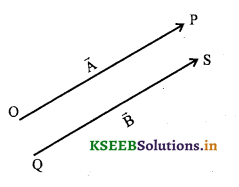Question 19.
What are parallel vectors?
Two vectors whose line of action are parallel are called parallel vectors.

Question 20.
What are like vectors?
Vectors which are parallel and having the same direction are called like vector.

Question 21.
What are unlike vectors?
Vectors which are parallel and having the opposite direction are called un-like vectors.

Question 22.
What are co-initial or concurrent vectors?
Vectors whose initial points or origins coincide are called co-initial vectors.

Question 23.
What are co-planar vectors?
Vectors lying in the same plane are called co-planar vectors.

Multiplication of Vectors by Real Numbers

Question 1.
What is multiplication of a vector by a real numbers? Illustrate it by some examples.
When a vector A is multiplied by a real numbers ‘λ’ we get another vector λ$$\overrightarrow{\mathrm{A}}$$ The magnitude of λ$$\overrightarrow{\mathrm{A}}$$ is A times the magnitude of $$\overrightarrow{\mathrm{A}}$$.
If λ is positive, then the direction of λ$$\overrightarrow{\mathrm{A}}$$ is same as that of $$\overrightarrow{\mathrm{A}}$$
If λ is negative, then the direction of λ$$\overrightarrow{\mathrm{A}}$$ is opposite to that of $$\overrightarrow{\mathrm{A}}$$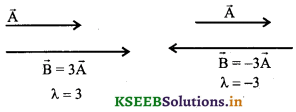Question 2.
What happens to magnitude and direction of a vector when it is multiplied by a (i) Positive number (ii) negative number?
(i) When a vector $$\vec{A}$$ is multiplied by a positive number λ, results is a vector whose magnitude is λA but the direction is same as that of $$\vec{A}$$.
(ii) When a vector A is multiplied by a negative number λ, it result in a vector whose magnitude is λA but its direction is opposite to that of $$\vec{A}$$.

Question 3.
Give an example for a vector multiplied by a scalar.
(i) $$\vec{F}=m \vec{a}$$
(ii) $$\vec{P}=m \vec{v}$$

Addition and Subtraction of Vectors-Graphical Method

Question 1.
Mention the two laws based on which the vectors can be added.
(i) Law of triangle of vectors
(ii) Law of parallelogram of vectors.

Question 2.
Describe the law of addition of two vectors by graphical method.
Consider two vectors $$\vec{A}$$ and $$\vec{B}$$ which lie is the same plane as shown in diagram (a). The lengths of the line segment of the two B vectors are proportional to the magnitude of the two vectors.
Now the vector $$\vec{B}$$ is placed such that its tail is at the head of the vector $$\vec{A}$$. Then the line joining the tail of $$\vec{A}$$ to the head of $$\overrightarrow{\mathrm{B}}$$ represents the vector $$\vec{R}$$ which is the sum of $$\vec{A}$$ and $$\vec{B}$$ shown in diagram (b)Question 3.
State the law of triangle of vectors.
If two vectors of same kind are represented by the magnitude and direction by the two sides of a triangle taken in order then their resultant is represented by the third side of the triangle taken in reverse order.

Question 4.
What do you mean by the statement the vector addition is commutative?
If $$\vec{A}$$ and $$\vec{B}$$ are two vectors, then according to commutative law $$\vec{A}+\vec{B}=\vec{B}+\vec{A}$$

Question 5.
What do you mean by the statement that the vector addition is associative?
If $$\vec{A}$$ , $$\vec{B}$$ and $$\vec{C}$$ are three vectors then according to associative law, $$\vec{A}(\vec{B}+\vec{C})=(\vec{A}+\vec{B})+\vec{C}$$

Question 6.
What is negative of a vector?
A vector equal in magnitude but opposite in direction to a given vector is called the negative of the given vector.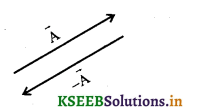Question 7.
What is the result of addition of two equal and opposite vectors?
Since the magnitude of two vectors are same but opposite in direction, the resultant vector has zero magnitude and is called null vector or zero vector.
$$\vec{A}-\vec{A}=\overrightarrow{0}$$

Question 8.
What is a zero or null vector?
A vector whose magnitude is zero is called a zero or null vector.

Question 9.
What is the magnitude and direction of null vector?
The magnitude of a null vector is zero and its direction cannot be specified.
$$|\vec{O}|=0$$

Question 10.
Mention the important properties of a null vector.
If $$\vec{A}$$ is the vector λ is the scalar thenQuestion 11.
What is the physical meaning of zero vector?
Consider an object at P at any instant r. Let the object mores to P’ and then comes back to P. Since the initial and final position is same. The displacement is a zero vector or null vector.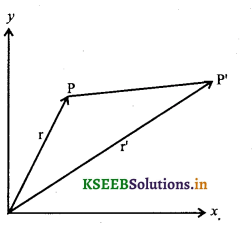Question 12.
Define subtraction of two vectors.
The subtraction of two vectors $$\vec{A}$$and $$\vec{B}$$ is defined as sum of the two vectors
$$\vec{A}$$ and $$\vec{-B}$$
i.e., $$\vec{A}-\vec{B}=\vec{A}+(-\vec{B})$$Question 13.
Show diagrammatically the subtraction of two vectors. Explain parallelogram method to find the sum (resultant) of two vectors.
Consider two vectors $$\vec{A}$$ and $$\vec{B}$$. To find their sum, the tails of the two vectors are brought to a common origin O. Then a line is drawn from head of $$\vec{A}$$ and parallel to $$\vec{B}$$. Another line is drawn from head of to $$\vec{b}$$ and parallel to $$\vec{A}$$ to complete the parallelogram OQSP. Now a line is drawn from the point of intersection to the origin O.
Thus the diagonal (OS) of the parallelogram represent the sum of the two vectors i.e., resultant of the two vectors.

Question 14.
State law of parallelogram of vectors.
If two vectors acting at a point are represented in magnitude and direction by the two adjacent sides of a parallelogram drawn from a point, then their resultant is represented both in magnitude and direction by the diagonal of the completed parallelogram drawn from the same point.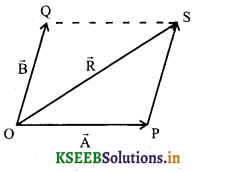Question 15.
Mention the expression for magnitude and direction of resultant of two vectors.
Let $$\vec{A}$$ and $$\vec{B}$$ are the two vectors and 0 be the angle between them. The magnitude of the resultant R is
R = $$\sqrt{A^{2}+B^{2}+2 A B \cos \theta}$$
If α is the angle made by resultant with the vector A then tan α = $$\frac{B \sin \theta}{A+B \cos \theta}$$
α = tan-1 $$\left[\frac{B \sin \theta}{A+B \cos \theta}\right]$$Resolution of Vectors

Question 1.
What is meant by resolution of a vector?
The process of splitting a vector into two or more component vectors such that the component vectors combine vectorially to produce the same effect as produced by the given vector is called resolution of a vector.

Question 2.
Explain the method of resolution of a vectors and have to find the component vectors.
-To find component of a vector $$\vec{A}$$ , consider two non-zero vector $$\vec{a}$$ and $$\vec{b}$$ such that the vectors are in the same plane.
Let O and P be the tail and head of the vector $$\vec{A}$$ Then through O draw a line parallel to $$\vec{a}$$ and through P draw a line parallel to $$\vec{b}$$ so that they intersect at Q.
From the diagram
OP = OQ + QP
v OQ is parallel to a, OQ = λ$$\vec{a}$$
Similarly QP is parallel to $$\vec{b}$$ , QP = μ$$\vec{b}$$
where λ and μ are real numbers
.’. A = λ$$\vec{a}$$ + μ$$\vec{b}$$
Now A is resolved into two component vector λ$$\vec{a}$$ and μ$$\vec{b}$$ along $$\vec{a}$$ and $$\vec{b}$$ respectively.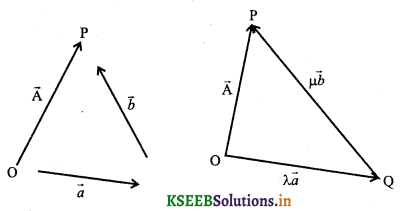Question 3.
What is a unit vector?
A vector of unit magnitude is called unit vector. It has no dimension and unit. It is used to specify direction only.

Question 4.
Give the mathematical representation of unit vector.
$$\widehat{A}=\frac{\vec{A}}{|\vec{A}|}$$

Question 5.
What are the rectangular components of a vector?
If a vector is resolved into components along two mutually perpendicular directions, then the components are called rectangular components.

Question 6.
Mention the unit vectors along x, y and z axes of rectangular co-ordinate system.
The unit vectors along x, y and z axes of a rectangular co-ordinate systems are i, j and k respectively.

Question 7.
Explain the method to express a vector $$\vec{A}$$ in terms of rectangular components in x-y plane.
Consider a vector $$\vec{A}$$ in the x-y plane. From the head of $$\vec{A}$$ draw perpendicular to co-ordinate axis to get vectorsQuestion 8.
Can the component of a vector be negative?
Yes, the component of a vector can be positive, negative or zero depending on the value of θ.

Question 9.
A vector $$\vec{A}$$ makes an angle θ with x-axis what are its components along x-axis and y-axis.
The component along x-axis is Ax = Acos θ
The component along y-axis is Ay = A sin θ

Question 10.
Calculate the magnitude and direction of a vector in terms of its components.
If Ax and Ay are the components of a vector along x-axis and y-axis respectively then
Ax = Acos θ …. (1)
Ay = A sin θ ….(2)
where θ is the angle made $$\vec{A}$$ with the x-axis squaring and adding (1) and (2)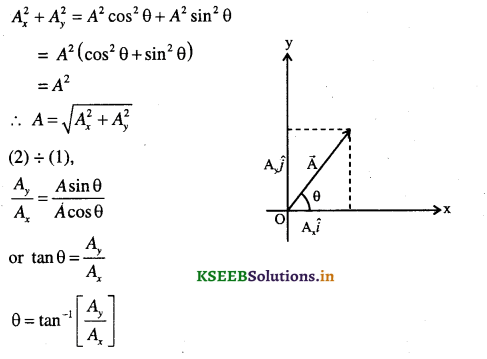Question 11.
Mention the components of a vector in three dimensions.
If α β and γ are the angles made by $$\vec{A}$$ with x, y and z axes respectively then
Ax – Acosα
Ay – Acosβ
Az = Acosγ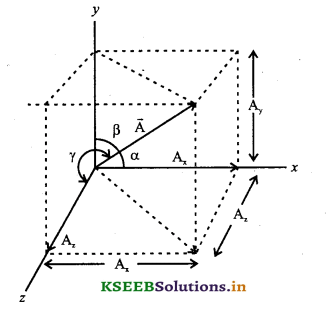Question 12.
Mention the expression for magnitude of a vector in terms of components in three dimensions.
The magnitude of a vector A is A = $$\sqrt{A_{x}^{2}+A_{y}^{2}+A_{z}^{2}}$$ where Ax,Ay and Az are the components of $$\vec{A}$$ along x-axis, y-axis and z-axis respectively.

Question 1.
Show that the component of the resultant of a vector is the sum of the corresponding components (by analytical method)
Consider two vectors $$\vec{A}$$ and $$\vec{B}$$ in the x-y plane with components Ax, Ay and Bx,By.∴ component of the resultant vector is the sum of the corresponding components of the vectors A and B .

Question 2.
Obtain an expression for magnitude and direction of the resultant of two vectors.
Expression for magnitude of the resultant:
Consider two vectors $$\vec{A}$$ and $$\vec{B}$$ acting at a point O. Let the two vectors $$\vec{A}$$ and $$\vec{B}$$ are represented by OP and OQ both in magnitude and direction. Let OS represents the resultant vector both in magnitude and direction.
Using law of parallelogram of vectors $$\vec{R}=\vec{A}+\vec{B}$$
Draw SN perpendicular to OP
From the right angled triangle ONS,
OS2 =ON2 +SN2
But ON = (OP + PN)
∴ OS2 =ON2 +SN2 ….. (1)
From the triangle PSN,
sin θ = $$\frac{S N}{P S}$$ => SN = PS sin θ
cos θ = $$\frac{P N}{P S}$$ => PN = PS cos θ
Substituting for SN and PN in (1)
OS2 = (OP + PS cosθ)2 + (PS sinθ)2
But OS = R, OP = A and PS = B
∴ R2 =(A + Bcosθ)2 +(Bsinθ)2
= A2 +2ABcosθ + B2cos2θ + B2sinθ
= A2 + 2ABcosθ + B2(cos2θ + sin2θ )
R2 = A2 + 2ABcosθ + B2
R = $$\sqrt{A^{2}+2 A B \cos \theta+B^{2}}$$ ….. (2)
Equation (2) represents the magnitude of the resultant.
To find the direction of the resultant:
From the triangle OSN
tan α =$$\frac{S N}{O N}=\frac{S N}{O P+P N}$$
But, SN = PS sin θ = B sin θ
PN = PS cos θ = B cos θ Bsinθ
∴ tan α =$$\frac{B \sin \theta}{A+B \cos \theta}$$
α =tan-1 $$\left[\frac{B \sin \theta}{A+B \cos \theta}\right]$$ …. (3)
Equation (3) represents the direction of the resultant.

Question 3.
Arrive at the law of sines.
Let $$\vec{A}$$ and $$\vec{B}$$ are two vectors acting at a point and $$\vec{R}$$ be their resultant.
From the triangle OSN,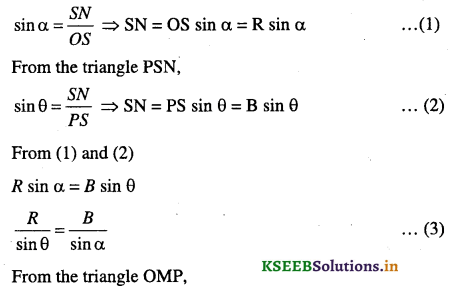This is known as law of sines.

Question 4.
When the magnitude of two vector of two vectors is (a) maximum (b) minimum.
(a) maximum when θ = 0, i.e., when two vectors are in the same line and same direction.
(b) minimum when θ = 180° i.e., when two vectors are in the same line but opposite direction.

Question 5.
What is the greatest and least resultant of two forces A and B?
Greatest resultant Rmax =A + B
Least resultant Rmin = A – BQuestion 6.
How can a vector be tripled?
By multiplying the vector oy 3 or by adding two similar vector to the given vector.

Question 7.
Can the velocity vector and displacement vector be added?
No, only similar vector cannot be added.

Question 8.
Two vectors $$\vec{A}$$ and $$\vec{B}$$ are acting at right angles to each other. What is the magnitude of their resultant?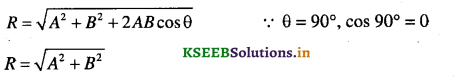Question 9.
What is the maximum number of components into which the vector can be resolved?
Infinity. A vector can be resolved into any number of components.

Question 10.
Can two vectors of same magnitude have resultant equal to either of them?
Yes. When the angle between them is 120°.

Motion in a Plane

Question 1.
A force is inclined at 30° to the horizontal. If its rectangular component in the horizontal direction be 50 N, find the magnitude of the force and its vertical component.
F cos θ = 50 givenF = 57.74 N
Vertical component = F sin θ
= 57.74 x sin 30°
= 57.74 x 1/2
= 28.87 N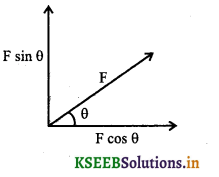Question 2.
One of the rectangular components of a velocity of 80 kmph 1 is 40 kmph-1. Find the other component.
V = 80 kmph-1
v2 = vx2 + vy2
Vy2 = v2 -Vx2
Vy = $$\sqrt{V-V_{x}^{2}}$$
= $$\sqrt{80^{2}-40^{2}}=\sqrt{4800}$$
= 69.28 kmph-1

Question 3.
Mention the expression for position vector of a particle located in a plane.
The position vector $$\vec{r}$$ of a particle located in a plane is $$\vec{r}=x \hat{i}+y \hat{j}$$
where x and y are the components of $$\vec{r}$$ along x and y – axes.

Question 4.
Give the pictorial representation of a position vector. Arrive at the displacement of a particle in the component form and hence represent the same pictorially.Let P and P’ be the position of the particle at instants t and t’ respectively. The position vector at these two positions be $$\vec{r}=x \hat{i}+y \hat{j}$$ and $$\vec{r}^{\prime}=x^{\prime} \hat{i}+y \hat{j}$$
Then displacement of the particle is $$\overrightarrow{\Delta r}=\vec{r}^{\prime}-\vec{r}$$
= (xî + yĵ ) – (xî + yĵ)
(x’ – x)î + (y’ – y)ĵ
Displacement $$\overrightarrow{\Delta r}=\Delta x \hat{i}+\Delta y \hat{j}$$Question 5.
Define average velocity and arrive at expression for the same vectorially.
The average velocity $$(\vec{v})$$ of a particle is defined as ratio of displacement of the particle to the corresponding time interval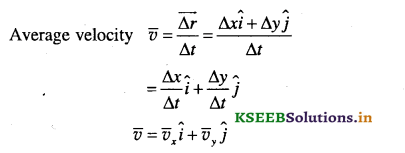Question 6.
What is the direction of average velocity?
The direction of average velocity of a particle is same as displacement of the particle.Question 7.
Define instantaneous velocity of a particle.
The instantaneous velocity of the particle is defined as limiting value of the average velocity as the time
interval approaches zero.
$$\vec{v}=\lim _{\Delta v \rightarrow 0} \frac{\Delta \vec{r}}{\Delta t}=\frac{d \vec{r}}{d t}$$

Question 8.
Arrive at the expression for average velocity in the component form and what is its direction?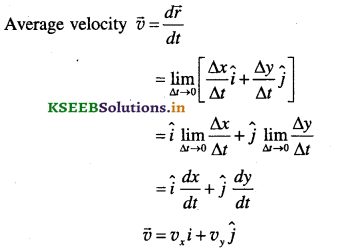The direction of velocity at any point on the path is the tangent to the path at that point.

Question 9.
Mention the expression for magnitude and direction of velocity in terms of its components along x axis andy axis.where vx and vy are the components of v along x-axis and y-axis and θ is the angle made by v with x axis.

Question 10.
Define average acceleration and mention its expression in the vector form.
The average acceleration of a particle for a time interval ∆t moving in x-y plane is the ratio of change in velocity to time interval.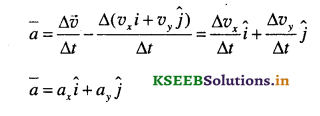Question 11.
Define instantaneous acceleration and mention the expression for the same.
The instantaneous acceleration is the limiting value of the average acceleration as the time interval approaches zero.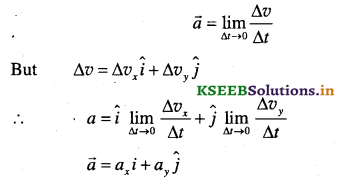Question 12.
What is the direction of velocity and acceleration in (i) one dimension (ii) two or three dimension?
(i) The velocity and acceleration of a particle are always along the same straight line (either in the sa’me direction or in the same direction).
(ii) The velocity and acceleration vectors may have any angle between 0° and 180° between them.

Question 13.
Show pictorially the angle between velocity (v) and acceleration (a) when the particle is moving in x-y plane.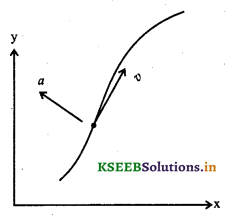Motion in a Plane with Constant Acceleration

Question 1.
Derive the equation v = v0 + at and hence express the same in terms of components along x-axis and y-axis.
Let v0 be the velocity of the particle at t = 0
v be the velocity at any instant t
a be the constant acceleration
from definition
a = $$\frac{v-v_{0}}{t-0}=\frac{v-v_{0}}{t}$$
or v – v0 = at
v = v0 + at
If vx and Vy be the component of velocity along x-axis and y-axis respectively,
vx = V0x + axt
vy= Voy + ayt

Question 2.
Derive the equation r = r0+v0t + $$\frac{1}{2}$$at2 and hence express the same in the component form.
Let r0 and r be the position vectors of the particle at O and t.
v0 and v be the velocity of the particle at O and t.
We know
displacement = (average velocity) (time)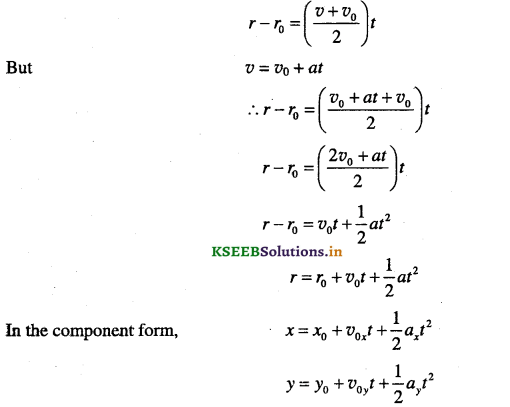Question 3.
Define relative velocity and mention the expression for the same.
When two bodies A and B are in motion the relative velocity of A with respect to B is defined as time rate of change of displacement of A with respect to B.
If VA and vB are the velocity of two bodies A and B respectively,
Velocity of A relative to B is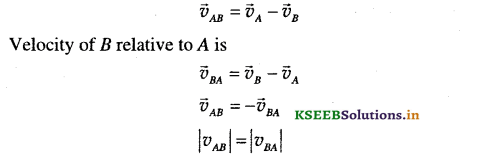Question 4.
What is two dimensional motion?
Motion of a particle in a plane is called two dimensional motion.

Projectile Motion

Projectile Motion Calculator is a free online tool that displays the motion of an object which is projected into the air.

Question 1.
What is a projectile?
An object that is flight after being thrown is called projectile.

Question 2.
Mention few examples for projectile.
(i) A cricket ball thrown up
(ii) A stone thrown from the ground
(iii) A football, baseball thrown up etc.

Question 3.
What happens to (i) horizontal component (ii) vertical component of velocity of a projectile during its motion
(i) The horizontal component of velocity of a projectile remains constant throughout its motion.
(ii) The vertical component of velocity is affected by the force of gravity.
Hence the horizontal motion is a uniform motion while the vertical motion is an accelerated motion with constant acceleration.

Question 4.
What is trajectory of a projectile?
The path traversed by a projectile is called trajectory.

Question 5.
For a projectile launched with a velocity v0, what is the components of (a) initial velocity (b) acceleration on it due to gravity
(a) vθx= v0 cosθ
voy =v0sinθ

(b) ax = 0
ay = -g

Question 6.
Mention the expression for x andy co-ordinate of the position of the projectile at any instant t in terms of initial speed (v0) and angle of projection θ.
x = v0xt = (v0cosθ)t
vy = (v0sinθ)t – $$\frac{1}{2}$$ gt2

Question 7.
Mention the expression for components of velocity at any instant H’ in terms of initial speed v0 and angle of projection θ
x = v0x = v0cosθ
vy = v0sinθ – $$\frac{1}{2}$$ gt

Question 8.
What is the value of component of velocity in the vertical direction at the point of maximum height?
The component of velocity in the vertical direction at the point of maximum height is vy = 0.

Question 9.
What is the trajectory of a projectile?
The curved path followed by a projectile is called trajectory.

Question 10.
What is the nature of trajectory of a projectile?
The trajectory of a projectile is a parabola.

Question 11.
Write the equation for the trajectory of a projectile?
The equation of the trajectory of the projectile is y = x tan θ – $$\frac{g}{2\left(v_{0} \cos \theta\right)^{2}} x^{2}$$

Question 12.
What is projectile? Show that the trajectory of a projectile is parabola.
Projectile is an object thrown up and allowed fall freely under gravity.
Consider a projectile thrown up from the point O. Let v0 be the initial velocity of the projectile at an angle 0 to the horizontal (positive x-axis).
This initial velocity v0 can be resolved into two components as vx – v0 cos θ along horizontal and vy = v0 sin θ along the vertical.
The horizontal component v0 cos θ remains constant throughout motion while the vertical component decreases and becomes zero at the highest point, distance travelled along ox in a time t is,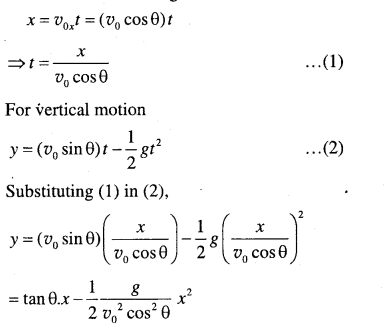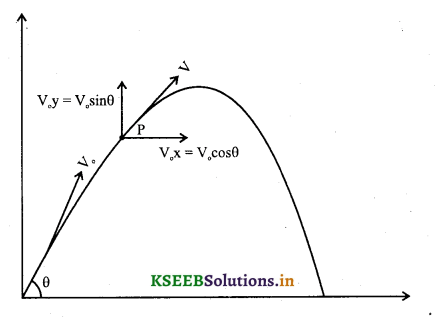Since θ, g and Vo are constants, the above equation can be written as
y = ax + bx2 …..(3)
a = tan θ , b = $$\frac{-g}{2 v_{0}^{2} \cos ^{2} \theta}$$
Equation (3) is the equation for parabola. Thus the path traded by a projectile is a parabola.

Question 13.
Define time of flight?
It is the time during which the projectile remains in flight.Question 14.
Derive an expression for time of flight?
In case of a projectile projected from the ground, time of flight is the time taken to reach the ground. It is the sum of time of ascent and time of descent,
time of flight = time of ascent + time of descent
Tf = ta + td
but ta = td
Tf = 2 ta
We know v = v0 + at
for vertical motion v0 = v0 sin θ
v = 0
a = -g, t – ta
∴ O = v0 sin 0 – g ta
ta = $$\left(\frac{v_{0} \sin \theta}{g}\right)$$
∴ time of flight Tf=2ta
= $$\left(\frac{2 v_{0} \sin \theta}{g}\right)$$

Question 15.
What is the angle of projection for which time of flight is maximum and mention the expression for the same?
The time of flight is maximum when θ = 90°
Tf = $$\frac{2 v_{0} \sin \theta}{g}$$
Tf is maximum when θ = 90°, sin 90° = 1
Tf max = $$\frac{2 v_{0}}{8}$$

Question 16.
Obtain an expression for maximum height attained by the projectile.
Maximum height is maximum distance travelled by the projectile in the vertical direction
x = v0t +$$\frac{1}{2}$$at2
for vertical motion,Question 17.
What is the angle of projection for which height attained by a projectile is maximum and mention the expression for the same?
hm = $$\frac{v_{0}^{2} \sin ^{2} \theta}{2 g}$$
The height attained is maximum when θ = 90°, sin 90° = 1
hmax = $$\frac{v_{0}^{2}}{g}$$

Question 18.
Define horizontal range of a projectile.
It is the maximum distance travelled by the horizontal along the projectile in a time equal to its time of flight.

Question 19.
Obtain an expression for range of a projectile. What is the angle of projection for which the range is maximum?
Range of a projectile is the distance travelled by the projectile in the horizontal direction in a time equal to time of flight with a constant velocity v0 cos θ
distance travelled = Velocity x time taken
(horizontal distance) = (horizontal velocity) (time of flight)Question 20.
Which component of velocity of a projectile remains constant?
Horizontal component of velocity.

Question 21.
Which component of velocity of a projectile is affected by gravity?
Vertical component of velocity.Question 22.
Which component of velocity of a projectile is zero at maximum height?
Vertical component of velocity.

Question 23.
What is value of horizon tal component of velocity at the maximum height?
vocos θ
where v0 is the initial velocity and 0 is the angle of projection.

Question 24.
Which component of acceleration of a projectile is zero?
Horizontal component

Question 25.
Draw the diagram for the path of the projectile. Indicate (a) angle of projection (b) maximum height .
(c) range of the projectile.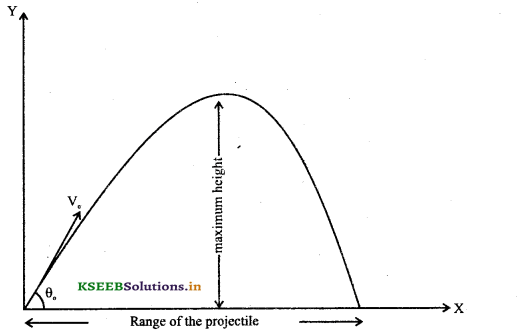Uniform Circular Motion

Question 1.
What Is uniform circular motion?
The motion of an object along a circular path at a constant speed is called uniform circular motion.

Question 2.
An object executing uniform circular motion has acceleration, though it moves with a constant speed. Explain?
Though the speed of the object remains constant, the direction changes continuously. Hence, the object has acceleration.

Question 3.
For a particle executing uniform circular motion the speed is uniform hut its velocity is not uniform explain.
The direction of velocity, given by the tangent changes at every point, Hence the velocity changes even though speed is constant.Question 4.
Show that acceleration of a particle executing uniform circular motion is along the radius and directed towards the centre of the circular path.
Let r and r’ be the position vectors and $$\vec{v}$$ and $$\vec{v}^{\prime}$$ the velocities of a particle at points P and P’ respectively. The velocity vectors $$\vec{v}$$ and $$\vec{v}^{\prime}$$ is given by tangents drawn P and P’. Further $$\vec{v}$$ is perpendicular to $$\vec{r}$$ and $$\vec{v}^{\prime}$$ is
perpendicular to $$\vec{r}^{\prime}$$. Hence, $$\Delta \vec{v}$$ is perpendicular to $$\Delta \vec{r}$$ The average acceleration $$\bar{a}=\frac{\Delta \vec{v}}{\Delta \vec{r}}$$ is along $$\Delta \vec{v}$$ , the
average acceleration is perpendicular to $$\Delta \vec{r}$$ . If $$\Delta \vec{v}$$ is on the line bisecting the angle between $$\vec{r}$$ and $$\vec{r}^{\prime}$$, the average acceleration is along the radius and directed towards the centre.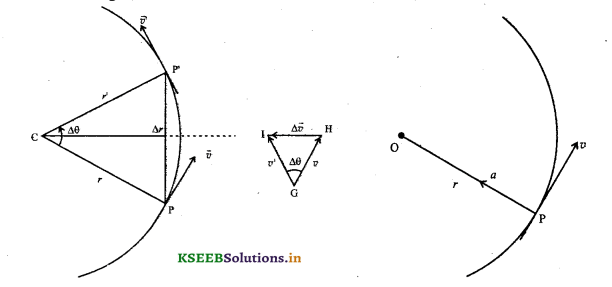Question 5.
Define centripetal acceleration.
The acceleration of particle executing uniform circular motion is called centripetal acceleration.

Question 6.
What is the direction of centripetal acceleration?
It is along the radius and directed towards the centre of the circular path.

Question 7.
Obtain an expression for centripetal acceleration?
Let r and r’ be the position vectors and $$\vec{v}$$ and $$\vec{v}^{\prime}$$ be the velocities of a particle at points P and P’.
Let the angle between r and r1 be ∆θ. The velocity vectors $$\vec{v}$$ and $$\vec{v}^{\prime}$$ are perpendicular to position vectors r and r’.
From similar triangle CPP’ and GHI the ratio of base length and side length are equal.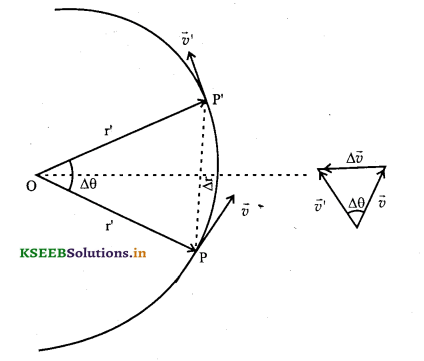Question 8.
Does the magnitude and direction of centripetal acceleration remains? Explain.
Centripetal acceleration a = $$\frac{v^{2}}{R}$$
Since the magnitude of velocity and the radius R remains constant, the magnitude of centripetal acceleration remains constant. However the direction changes but always directed towards the centre of the circular path.

Question 9.
Define angular speed?
Angular speed of a particle executing circular motion is defined as the time rate of change of angular displacement.
w =$$\frac{\Delta \theta}{\Delta t}$$
Where ∆θ is the angular distance, ∆t is the time takenQuestion 10.
Obtain the relation between linear speed (y) and angular speed (ω).
Let a particle move from P to P’ covering an angular distance AO and
linear distance ∆s in a time ∆t.
Angular speed = $$\frac{\Delta \theta}{\Delta t}$$
But linear distance y = $$\frac{\text { distance travelled }}{\text { time taken }}$$
v = $$\frac{\Delta s}{\Delta t}$$
But ∆s=R∆θ (∴ S=rθ]
v = R $$\frac{\Delta \theta}{\Delta t}$$
y = R.ωQuestion 11.
Mention the expression for centripetal acceleration in terms of linear speed and express it in terms angular speed.
Centripetal acceleration a = $$\frac{v^{2}}{R}$$
But v = Rω
∴ a = $$\frac{(R \omega)^{2}}{R}$$ = ω2R

Question 12.
Define (a) time period (b) frequency of particle executing uniform circular motion.
The time taken by the particle to complete one revolution is known as time period.
The number of revolutions made by the particle in one second is known frequency.

Question 13.
Express the expression for centripetal acceleration in terms of (a) time period (b) frequency of revolution.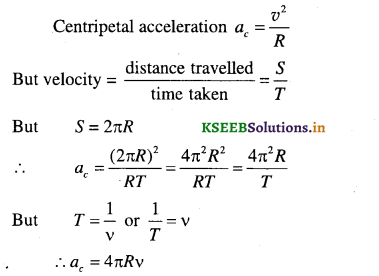Numerical Problems

Question 1.
An arrow is shot into air with an initial velocity 20 ms-1 at angle 30° with the horizontal. Calculate
(a) Maximum height attained by the arrow (b) Range of the arrow.
v0 =20 ms-1, θ = 30°, h = 2, R = ?Question 2.
A body is projected up with an initial velocity of 25 ms-1 at an angle 35° with the horizontal. Calculate
(a) Maximum height (b) time taken to reach the maximum height (c) horizontal range.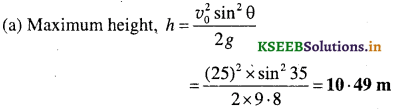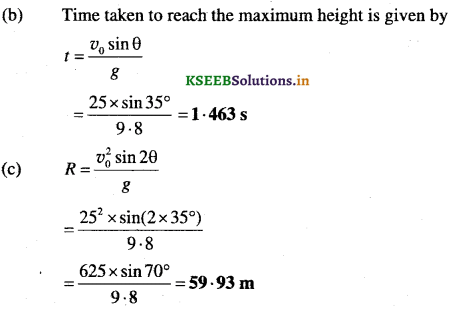Question 3.
An aeroplane flying horizontally at a speed of 324 km h-1 and a height 1.96 km releases a bomb. Find (a) Time taken by the bomb to hit the ground, (b) How far the bomb will fall on the ground from the point of release (c) The magnitude and direction of velocity when the bomb hits the ground.
(a) Initial horizontal velocity v = 324 kmh-1 = $$\frac{324 \times 5}{18}$$ = 90 ms-1
Initial vertical velocity vy = 0, x = 1960
x = vyt + $$\frac{1}{2}$$ gt2
1960 = 0 + $$\frac{1}{2}$$ x 9.8 x t2
t = $$\sqrt{\frac{2 \times 1960}{9 \cdot 8}}$$

(b) Horizontal distance between the point of release and die point at which die bomb hits the ground is
Horizontal distance = vx x t
x = 90 x 20 = 1800 m

(c) During die motion horizontal component remains the same while the vertical component increases.
∴ vx = 90 ms-1
vy = v0 + gt
= 0 + 9.8 x 20
vy = 196 ms-1
The magnitude of the velocity with which it hits the ground is v = ^jv2x+z? =s/902+1962 .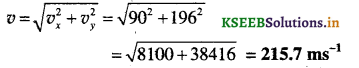If θ is the angle made by the resultant velocity with the horizontal their v
tanθ = $$\frac{v_{y}}{v_{x}}$$
= $$\frac{196}{90}$$ = 2.1777
θ = tan-1 2.1777 = 65°20′

Question 4.
A boy kicks a football at an angle of 30° to the ground so that maximum height of 4 m is reached. Calculate (a) Velocity of projection (b) time taken to reach the ground from the instant it is kicked
(c) Velocity with which the ball hits the ground.
(a) θ = 30°, hm= 4 m(b) Time taken to reach the ground(c) Velocity with which it hits the ground = velocity of projection = 17.71 ms-1Question 5.
State for each of the following physical quantities, if it is a scalar or a vector: Volume, mass, speed, acceleration, density, number of moles, velocity, angular frequency, displacement, angular velocity.
Volume – Scalar
Mass – Scalar
Speed – Scalar
Acceleration – Vector
Density – Scalar
Number of moles – Scalar
Velocity – Vector
Angular frequency – Scalar
Displacement – Vector
Angular velocity – Vector

Question 6.
Pick out the two scalar quantities in the following list: force, angular momentum, work, current, linear
momentum, electric field, average velocity, magnetic moment, relative velocity.
The two scalar quantities in the following list are work and current.

Question 7.
Pick out the only vector quantity in the following list: Temperature, pressure, impulse, time, power, total path length, energy, gravitational potential, co-efficient of friction, charge
The only vector in the list is impulse.

Question 8.
State with reasons, whether the following algebraic operations with scalar and vector physical quantities are meaningful, (a) adding any two scalars (b) adding a scalar to a vector of the same dimensions, (c) multiplying any vector by a any scalar (f) multiplying any two scalars (e) adding any two vectors (f) adding a component of a vector to the same vector.
(a) No, only vectors of same kind can be added.
(b) No. a scalar cannot be added to a vector.
(c) Yes, a vector can be multiplied by a scalar. Ex: F = ma, mass is scalar while acceleration is a vector quantity.
(d) Yes. two scalar can be multiplied work done = Power x time W = pt. Power and time are scalar
(f) No, component of vector cannot be added to the same vector.

Question 9.
Read each statement below carefully and state with reasons, if it is true or false: (a) The magnitude of a vector is always a scalar, (b) Each component of a vector is always a scalar, (c) The total path length is always equal to the magnitude of the displacement vector of a particle, (d) The average speed of a particle (defined as total path length divided by the time taken to cover the path) is either greater or equal to the magnitude of average velocity of the particle over the same interval of time, (e) Three vectors not lying in a plane can never add up to give a null vector.
(a) True, the magnitude of a vector is a pure number. Therefore it is always a scalar.
(b) False, the component of a vector is also a vector.
(c) False, the total path length is always greater than or equal to the magnitude of the displacement vector of the particle.
(d) True, average speed = $$\frac{\text { total path length }}{\text { time taken }}$$
and average velocity = $$\frac{\text { displacement }}{\text { time taken }}$$
Since total path length is greater than or equal to displacement, average speed is greater than or equal to average velocity.
(e) True, when the resultant of any two vector is equal and opposite to the third vector, it leads to a null vector only if all the three vectors are in the same plane.Question 10.
Given a + b + c+ d = 0, which of the following statements are correct: (a) a, b, c and d must be a null vector, (b) The magnitude of (a + c) equals the magnitude of (b + d) (c) The magnitude of a can Ps never be greater than the sum of the magnitude of b, c and d. (d) b + c must lie in the plane of a and d if a and d are not collinear, and the in the line of a and d. If they are not collinear?
(a) Not correct. Because a + b + c + d can be zero in many ways. The sum is zero even if a, b, c and d are not null vectors.

(b) Correct. The magnitude of (a + c) = (b + d)
a + b + c + d = 0 ⇒ (a + c) = – (b + d) ⇒ |(a + c)| = |(b + d)|

(c) Correct.
a + b + c + d = 0 ⇒ a = -(b + c + d) ⇒ |a| = |(b + c + d)|
The magnitude of a can never be greater than the sum of the magnitudes of b, c and d.

(d) Correct
a + b + c + d = 0=>(b + c) + a + d = 0
The resultant of b + c, a and d is zero only if b + c is in the plane of a and d.
If a and d are collinear, b + c must be in the plane of a and d.

Question 11.
Three girls skating on a circular ice ground of radius 200 m start from a point P on the edge of the ground and reach a point Q diametrically opposite to P following different paths as shown in the diagram. What is the magnitude of the displacement vector for each? For which girls is this equal to the actual length of path skated?
The magnitude of displacement is same for all the three girls ABC. The magnitude of displacement = diameter of the circular ground = 2 x 200 = 400 m.
The displacement vector is equal to the actual length of the path skated only for the girl B.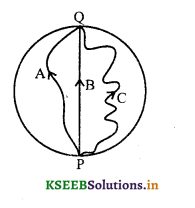Question 12.
A cyclist starts from the centre O of a circular park of radius 1 km, reaches the edge P of the park, then cycles along the circumference, and returns to the centre along QO as shown in the diagram. If the round trip takes 10 minute, what is the (a) net displacement (b) average velocity, and (c) average speed of the cyclist?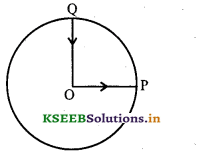(a) Since the final and initial position of the cyclist is same, net displacement is zero.Question 13.
On an open ground, a motorist follows a track that turns to his left by an angle of 60° after every 500 m. Starting from the given turn, specify the displacement of the motorist at the third, sixth and eighth turn. Compare the magnitude of the displacement with the total path length covered by the motorist in each case.
Since the motorist turns by an angle of 60° after every 500 m. The path followed by him is a regular hexagon as shown.
At the third turn,
Displacement = AD = (AG + GH + HD)
cos 60° = $$\frac{\mathrm{AG}}{\mathrm{AB}}$$ ⇒ AG = AB cos 60° = 500 x $$\frac{1}{2}$$ = 250 m
GH = BC = 500 m
cos 60° = $$\frac{\mathrm{HD}}{\mathrm{CD}}$$ ⇒ HD = CD cos 60° = 500 x $$\frac{1}{2}$$ = 250∴ Displacement AD = 250 + 500 + 250 = 1000 m
Path length = AB + BC + CD = 500 + 500 + 500 = 1500 m
$$\frac{\text { displacement }}{\text { path length }}=\frac{1000}{1500}=\frac{2}{3}$$
At the sixth turn :
At the sixth turn, the motorist reaches the initial position i.e., A.
∴ the displacement is zero.
The path length = AB + BC + CD + DE + EF + FA
= 500 + 500 + 500 + 500 + 500 + 500 = 3000 m
$$\frac{\text { displacement }}{\text { path length }}=\frac{0}{3000}=0$$

At the eighth turn :
At the eighth turn, the motorist will be at C.The direction of the displacement along AC making an angle 30° with the initial direction.
The path length = AB + BC + CD + DE + EF + FA 4- AB + BC
= 500 + 500 + 500 4 500 + 500 4 500 4 500 = 4000 mQuestion 14.
A passenger arriving in a new town wishes to go from the station to a hotel located 10 km away on a straight road from the station. A dishonest cabman takes him along a circuitous path 23 km long and reaches the hotel in 28 minute. What is (a) the average speed of the taxi (b) the magnitude of average velocity? are they equal?∴ Average speed is greater than the magnitude of average velocity.

Question 15.
Rain is falling vertically with a speed of 30 ms-1. A woman rides a bicycle with a speed of 10 ms-1 in the north to south direction. What is the direction in which she should hold the umbrella?
Let vr – velocity of the rain vertical downwards.
Vb – velocity of the bicycle in the north to south direction.
v = resultant velocity of the rain
To protect from the rain, the woman should hold the umbrella in the direction of v
From the diagram
tan θ = $$\frac{B C}{O B}=\frac{O A}{O B}=\frac{v_{b}}{v_{r}}$$
tan θ = $$\frac{10}{30}=\frac{1}{3}$$ = 0.3333
θ = tan-1 (0.3333) = 18°25′
∴ Woman should hold the umbrella at an angle 18°25′ with the vertical towards south.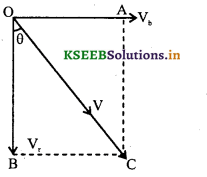Question 16.
A man can swim with a speed of 4 km hr-1 in still water. How long does he take to cross the river 1 km wide if the river flows steadily at 3 km hr-1 and he makes his strokes normal to the river current? How far down the river does he go when he reaches the other bank?
Let vm → Velocity of the man in still water = 4 km hr-1
vr → Velocity of the river = 3 km hr-1
v → Resultant velocity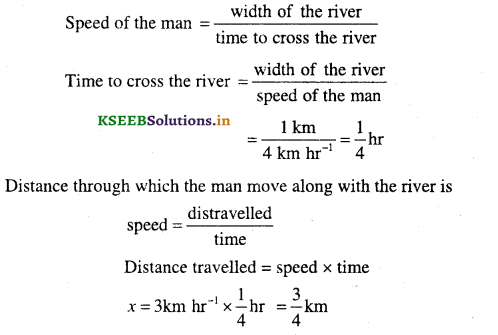Question 17.
In a harbour, wind is blowing at the speed of 72 km hr-1 and the flag on the mast of a boat anchored in the harbour flutters along the N-E direction. If the boat starts moving at a speed of 51 km hr-1 to the north, what is direction of the flag on the mast of the boat?
Since the flag flutters along N-E direction, velocity of wind vw = 72 km hr-1 along NE – direction velocity of the boat, v0 = 51 km hr-1.
vw → velocity of the wind
vb → velocity of the boat
vwb → relative velocity of wind with respect to the boat.
$$\vec{v}_{w b}=\vec{v}_{w}-\vec{v}_{b}=\vec{v}_{w}+\left(-\vec{v}_{b}\right)$$
The flag will flutter along the direction of vwb making an angle of α with -vb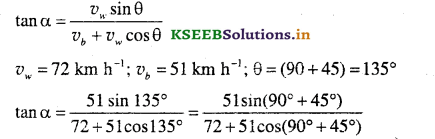tanα = 1
α = 45°
∴ The flag will be along the east.

Question 18.
The ceiling of a long hall is 25 m high. What is the maximum horizontal distance that a ball thrown with a speed of 40 ms-1 can go without hitting the ceiling of the hall?
h = 25 m, v0 = 40 ms-1, R = ?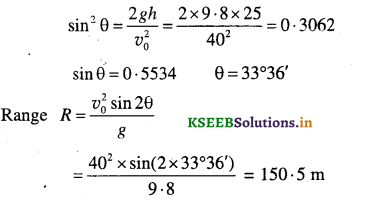Question 19.
A cricketer can throw a ball to a maximum horizontal distance of 100 m. How much high above the ground can the cricketer throw the same ball?
Rmax= 100 m
The Range R = $$\frac{v_{0}^{2} \sin 2 \theta}{g}$$
For maximum range sin 2θ = 1
∴ Rmax = $$\frac{v_{0}^{2}}{g}$$
v02 = Rmax.g = 100 g
For vertical motion,
v2 = v02 + 2 gh
v = 0,v0 = √100g, g = -9.8 ms-2, h – ?
0 = 100 g-2 x 9 – 8 x h
2 x 9.8 x h = 100 x 9.8
h = $$\frac{100 \times 9 \cdot 8}{2 \times 9 \cdot 8}$$ = 50 mQuestion 20.
A stone tied to the end of a string 80 cm long is whirled in a horizontal circle with a constant speed. If the stone makes 14 revolutions in 25 s. What is the magnitude and direction of acceleration of the stone?
r = 0.8 m, n = 14 revolutions
angular acceleration ac = ω2r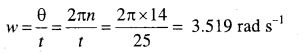∴ ac = ω2r
= 3.5192 x 0.8 = 9.90 ms-2Question 21.
An aircraft executes a horizontal loop of radius 1 km with a steady speed of 900 km h_1. Compare its
centripetal acceleration with the acceleration due to gravity.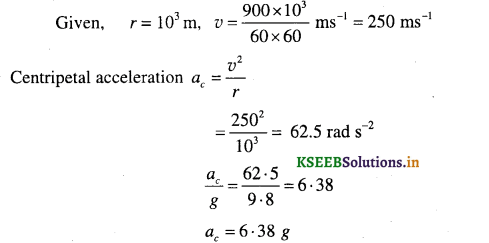Question 22.
Read each statement below carefully and state with reasons, if it is true or false:
(a) The net acceleration of a particle in circular motion is always along the radius of the circle towards the centre.
(b) The velocity vector of a particle at a point is always along the tangent to the path of the particle at that point.
(c) The acceleration vector of a particle in uniform circular motion averaged over one cycle is a null vector.
(a) False, because the net acceleration of the particle is towards the centre only if the particle is executing circular motion with a constant speed.
(b) True, this velocity vector is always along the tangent when the particle is in circular motion.
(c) True, because the displacement of the particle is zero in one complete cycle. Consequently the average acceleration over a compute cycle is also zero.

Question 23.
The position of a particle is given by $$\vec{r}$$ = 3 0 t î – 2.0t2 ĵ + 4.0k̂m where t is in seconds and the co-efficients have the proper units for r to be in metres.
(a) Find the $$\vec{v}$$ and $$\vec{a}$$ of the particle?
(b) What is the magnitude and direction of velocity of the particle at t = 2.0 s?If θ is the angle made by v with the x-axis then,
tan θ = $$\frac{v_{y}}{v_{x}}=\frac{-8}{3}$$ = -2.667 = 69°26′
∴ Velocity vector $$\vec{v}$$ makes an angle θ = 69°26′ with the x-axis.Question 24.
A particle starts from the origin at t = 0 s with a velocity of 10-0 ĵ ms and moves in the X-Y plane
with a constant acceleration of (8.0î + 2.0 ĵ ) ms-2 (a) At what time is the X-co-ordinate of the particle 16 m? What is the y-coordinate of the particle at that time? (b) What is the speed of the particle at that time?
The position of the particle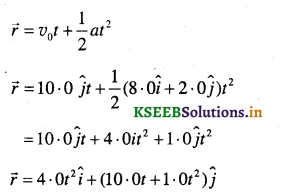∴ The component of r along x-axis is 4.0t2 and component of r along y – axis is (10.0t + 1.0t2)
x = 4t2 and y = 10t + t2
Given x = 16 m,
∴ 16 = 4t2 ⇒ r = 2s
The value of y-coordinate when t = 2s is y
= 10 x 2 + 4 = 24m

(b) Velocity of the particle at any instant t is
v = v0 + at
v = 10.0ĵt + (8.0î + 2.0ĵ)t
v = 8.0ĵt + (10.0 + 2-0t)ĵ
When t = 2s
v = 8.02î + (10.0 + 2.0 x 2)ĵ
= 16.0î + 14.0ĵ)
The magnitude of velocity